Earn 20 XP

## Learning Objectives

• Dependent and Independent Variables
• Equation of a Straight Line
• Linear Regression
• Cost

## Dependent and Independent Variables

• So far, you've been studying input and output/target variables. Commonly, the input variable is known as the independent variable, and the target variable is known as the dependent variable.
• In a nutshell, our target variable is nothing but a dependent variable. Why dependent? Because the values of this variable are dependent on other variables (i.e., input variables)
• And, our input variables are known as independent variables. Here the values of these variables are not dependent on any other variables.

• Remember the Standard Metropolitan Areas Data used previously? In that dataset, we might be curious to predict "crime_rate" in future, so that becomes our target variable (dependent variable) and the rest of the variables become input variables (independent variables) for building a machine learning model.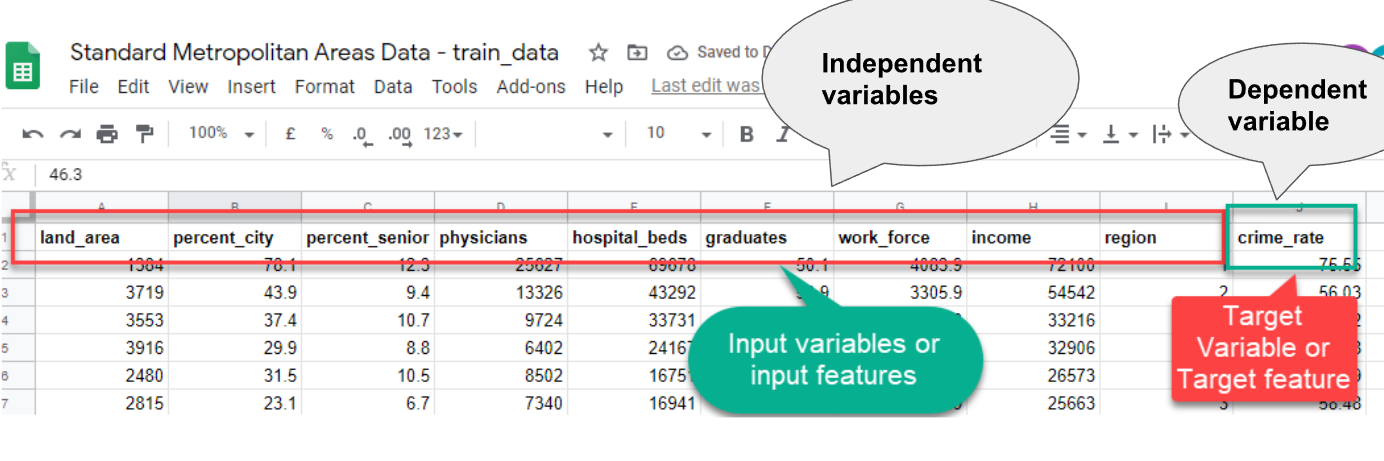### Another Example

• For example, a scientist wants to see if the brightness of light affects a moth being attracted to it.
• The scientist controls the brightness of the light. This would be the independent variable.
• The dependent variable would be how the moth reacts to the different light levels (distance to the light source).

### Equation of a Straight Line

• In algebra, a linear equation (equation of a straight line) typically takes the form y = mx + b, where m and b are constants, x is the independent variable, and y is the dependent variable.
• Basically, the value of y is calculated using x, whereas x has no dependence on the value of y.
• y = how far up
x = how far along
m = Slope or Gradient (how steep the line is)
b = value of y when x=0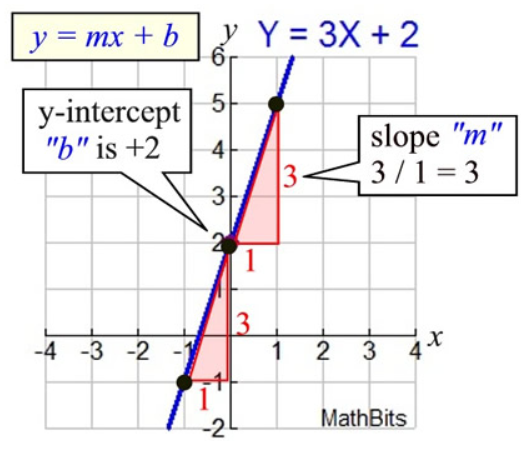• How do you find "m" and "b"?
• b is easy: see where the line crosses the Y axis.
• m (the Slope) needs some calculation: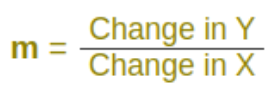### Synonyms Recap

Too many synonyms to memorize? Let me put them all down in one place for better understanding:

• Variables = Features
• Input Variables = Attributes = Predictor = Independent Variables
• Target Variables = Labels = Outcomes = Dependent Variables

## What is linear regression?

• Suppose you are thinking of selling your home. And various houses around you with different sizes (area in sq. ft) around you have sold for different prices as listed below: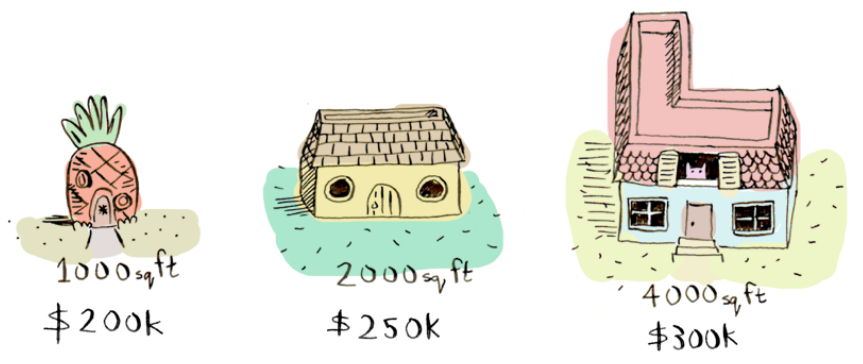• And considering, your home is 3000 square feet. How much should you sell it for?
• When you look at the existing price patterns (data) and predict a price for your home, that is an example of linear regression.
• Here's an easy way to do it. Plotting the 3 data points (information about previous homes) we have so far: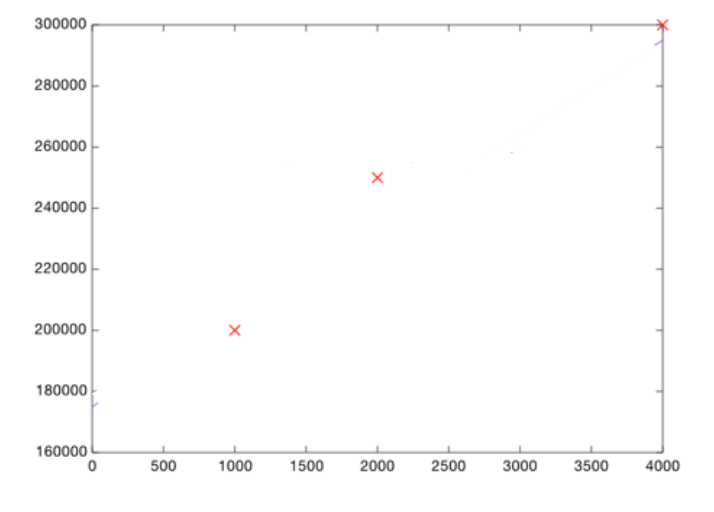• Now, you can eyeball it and roughly draw a line that gets pretty close to all of these points. Then look at the price shown by the line, where the square footage is 3000: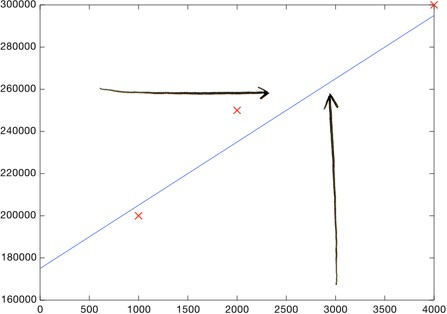• Boom! Your home should sell for \$260,000.
• That's all! (well, kind of) You plot your data, make a rough line, and use the line to make predictions. You need to make sure your line fits the data well (but not too well :)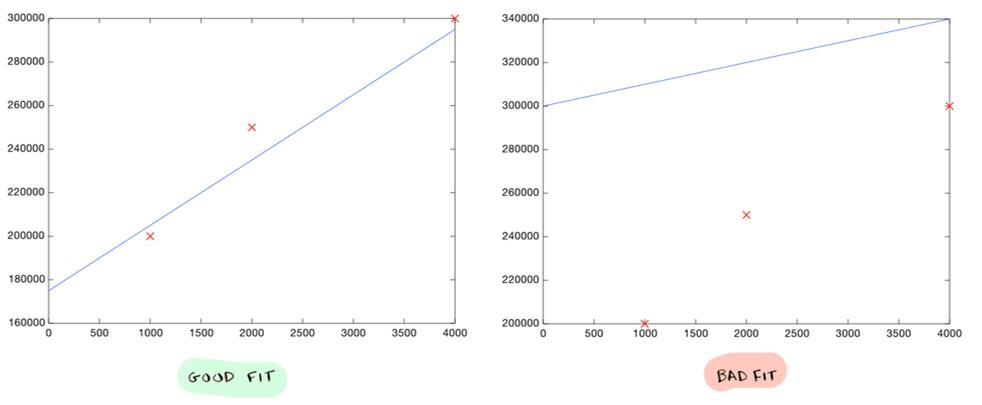But, of course, we don't want to make a line roughly. We want to compute the exact line that best "fits" our data. That's where machine learning comes into play!

### What is linear regression? - to summarize

• Linear regression is a linear model, i.e., a model that assumes a linear relationship (straight-line relationship) between the input variables (x) and the single output variable (y).
• When there is a single input variable (x), the method is referred to as simple linear regression or just linear regression. Eg: Salary dataset given here. There is only one target variable and one input variable where we are predicting the salary of individual using their years of experience.
• When there are multiple input variables, it is often referred to as multiple linear regression. Eg: Smart Metropolitan areas data set, we have multiple input variables.

### Which line is good?

• How do you decide what line is good? Here's a bad line: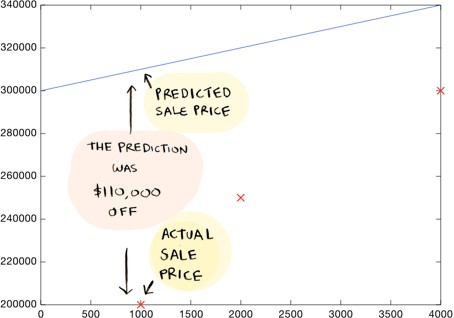• This above-drawn line is way oﬀ. For example, according to the line, a 1000 sq foot house should sell for \$310,000, whereas we know it actually sold for \$200,000.

### Here's a better line: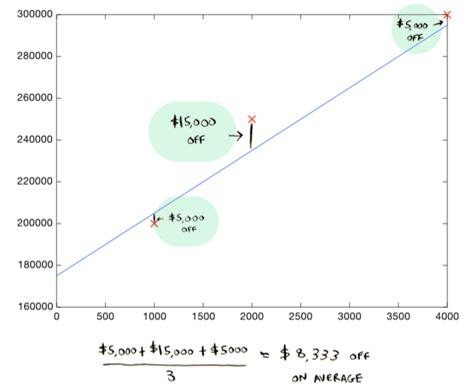• In this case, the residuals (absolute diﬀerence between the actual and the predicted value) are \$5000, \$15000, and \$5000.
• This line is an average of \$8,333 dollars oﬀ (adding all the distances and dividing by 3).
• This \$8,333 is the cost of using this line.

### Cost

• The cost is how far oﬀ the learned line is from the real data. The best line is the one that is the least oﬀ from the actual data.
• To find the best line (to find the values of coeﬃcients), we need to define and use a cost function.
• In ML, cost functions are used to estimate how badly models are performing.
• Put simply, a cost function measures how wrong the model is in estimating the relationship between X and y.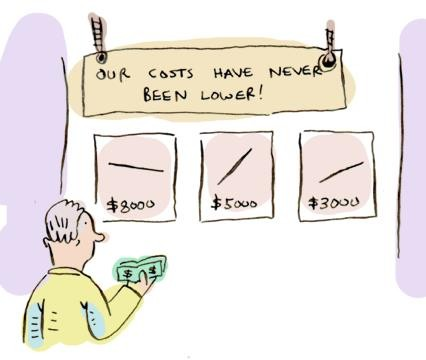### Cost Function

• There is also something called a Cost function that is associated with the analysis. It is slightly mathematical, and we will learn more about it soon!
• Meanwhile, let's run a simple linear regression model. The link to the notebook is given below.